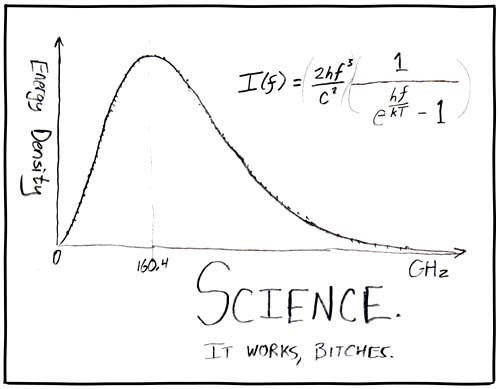## March 13, 2007

### CMB - it works, bitchesThe above diagram is the graph of energy density vs frequency for the Cosmic Microwave Background (CMB). One way to interpret it as a graph of how many photons per frequency were detected coming from CMB. The curve is called a Planck curve, which is described by the equation shown. So, how is this significant? Planck curves are seen when measuring radiation given off by a black body. Measurements of CMB show that the universe is a black body exactly as predicted by the Big Bang theory.

But wait, there is more! Below is a map of CMB:What can we tell from this? Firstly, if we picked any point on the above map and measured CMB as seen from that point we find that it is the same everywhere to 1 part in about 100,000. That is, CMB is uniform from any view point down to resolution of 1/100,000, as predicted by the Big Bang Theory.

So CMB provides some pretty good evidence for the Big Bang theory, what else can it do? Well, it can tell us the shape of our universe too.

There are 3 possible configurations for the universe, as illustrated below:The configurations are:

1. Closed - the universe is sphere like, with positive curvature. Think of the universe as if its wrapped around something completely.

2. Open - the universe is like a saddle, with negative curvature. This configuration, because of its negative curvature, can never wrap around anything completely.

3. Flat - the universe is flat, like a piece of paper, with no curvature.

So how can CMB tell us which configuration our universe most closely resembles? We do this by looking at the map of CMB and measure the size of the brightest spots. But how does this work? Well, the path light takes through space is determined by the curvature of space-time - in other words the configuration our universe is in. The follow diagrams are my poor attempts to illustrate the concept:The diagram shows the light paths for a flat universe, follow by an open universe, then finally a closed universe. Note how despite the origins of the light paths are the same, the black bar which represent the perceived size of objects are different. Theory predicts for a flat universe the size of brightest spots are ~1 degree across, ~0.5 degree for an open universe and ~1.5 degrees for a closed universe. With this knowledge and measurement of CMB, we can thus determine the configuration of the universe - which is flat to with in 2% margin of error!

Now that was cool, but the next thing CMB tell us as a consequence of the above is even cooler - there exists negative energy! Now why is that? Well, the expansion of the universe is influenced by momentum of the initial expansion, and gravity. When gravity is stronger than momentum of the initial expansion, the universe starts curving back on itself as gravity deforms space time - giving us a closed universe configuration. If momentum is greater then we have an open universe and finally, when gravity and momentum is in balance, we have a flat universe - which CMB data suggests that we do. Yet despite this, we are still measuring increased rate of expansion! This mean something is making the universe expand, and its not the initial momentum of expansion. This is evidence in support of the existence of negative energy which is working to expand the universe.

CMB - it works, bitches.
Steve